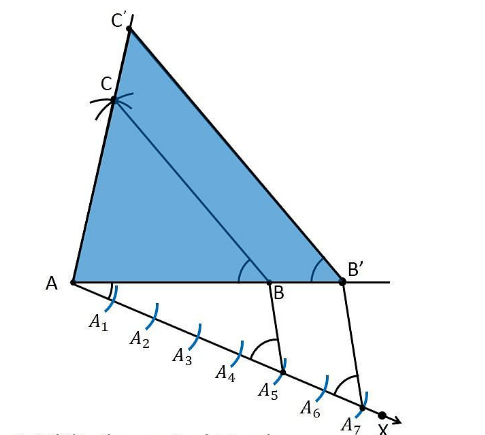Guru

# Construct a triangle with sides 5 cm, 6 cm and 7 cm and then another triangle whose sides are 7/5 of the corresponding sides of the first triangle Q.3

• 0

Sir please give me the best and simple way to solve the problem of question no.3 of exercise 11.1 of class 10th ncert chapter of question no.3, how i solve this important question  Construct a triangle with sides 5 cm, 6 cm and 7 cm and then another triangle whose sides are 7/5 of the corresponding sides of the first triangle

Share

1. Construction Procedure:

1. Draw a line segment AB =5 cm.

2. Take A and B as centre, and draw the arcs of radius 6 cm and 5 cm respectively.

3. These arcs will intersect each other at point C and therefore ΔABC is the required triangle with the length of sides as 5 cm, 6 cm, and 7 cm respectively.

4. Draw a ray AX which makes an acute angle with the line segment AB on the opposite side of vertex C.

5. Locate the 7 points such as A1, A2, A3, A4, A5, A6, A7 (as 7 is greater between 5 and 7), on line AX such that it becomes AA1 = A1A2 = A2A3 = A3A4 = A4A5 = A5A6 = A6A7

6. Join the points BA5 and draw a line from A7 to BA5 which is parallel to the line BA5 where it intersects the extended line segment AB at point B’.

7. Now, draw a line from B’ the extended line segment AC at C’ which is parallel to the line BC and it intersects to make a triangle.

8. Therefore, ΔAB’C’ is the required triangle.Justification:

The construction of the given problem can be justified by proving that

AB’ = (7/5)AB

B’C’ = (7/5)BC

AC’= (7/5)AC

From the construction, we get B’C’ || BC

∴ ∠AB’C’ = ∠ABC (Corresponding angles)

In ΔAB’C’ and ΔABC,

∠ABC = ∠AB’C (Proved above)

∠BAC = ∠B’AC’ (Common)

∴ ΔAB’C’ ∼ ΔABC (From AA similarity criterion)

Therefore, AB’/AB = B’C’/BC= AC’/AC …. (1)

In ΔAA7B’ and ΔAA5B,

∠A7AB’=∠A5AB (Common)

From the corresponding angles, we get,

∠A A7B’=∠A A5B

Therefore, from the AA similarity criterion, we obtain

ΔA A2B’ and A A3B

So, AB’/AB = AA5/AA7

Therefore, AB /AB’ = 5/7 ……. (2)

From the equations (1) and (2), we get

AB’/AB = B’C’/BC = AC’/ AC = 7/5

This can be written as

AB’ = (7/5)AB

B’C’ = (7/5)BC

AC’= (7/5)AC

Hence, justified.

• 0